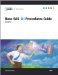# Chapter 2: The FREQ Procedure

## Overview

The FREQ procedure produces one-way to n -way frequency and crosstabulation (contingency) tables. For two-way tables, PROC FREQ computes tests and measures of association. For n -way tables, PROC FREQ does stratified analysis, computing statistics within, as well as across, strata. Frequencies and statistics can also be output to SAS data sets.

For one-way frequency tables, PROC FREQ can compute statistics to test for equal proportions, specified proportions , or the binomial proportion. For contingency tables, PROC FREQ can compute various statistics to examine the relationships between two classification variables adjusting for any stratification variables . PROC FREQ automatically displays the output in a report and can also save the output in a SAS data set.

For some pairs of variables, you may want to examine the existence or the strength of any association between the variables. To determine if an association exists, chisquare tests are computed. To estimate the strength of an association, PROC FREQ computes measures of association that tend to be close to zero when there is no association and close to the maximum (or minimum) value when there is perfect association. The statistics for contingency tables include

• chi-square tests and measures

• measures of association

• risks (binomial proportions) and risk differences for 2 — 2 tables

• odds ratios and relative risks for 2 — 2 tables

• tests for trend

• tests and measures of agreement

• Cochran-Mantel-Haenszel statistics

PROC FREQ computes asymptotic standard errors, confidence intervals, and tests for measures of association and measures of agreement. Exact p -values and confidence intervals are available for various test statistics and measures. PROC FREQ also performs stratified analyses that compute statistics within, as well as across, strata for n -way tables. The statistics include Cochran-Mantel-Haenszel statistics and measures of agreement.

In choosing measures of association to use in analyzing a two-way table, you should consider the study design (which indicates whether the row and column variables are dependent or independent), the measurement scale of the variables (nominal, ordinal, or interval), the type of association that each measure is designed to detect, and any assumptions required for valid interpretation of a measure. You should exercise care in selecting measures that are appropriate for your data.

Similar comments apply to the choice and interpretation of the test statistics. For example, the Mantel-Haenszel chi-square statistic requires an ordinal scale for both variables and is designed to detect a linear association. The Pearson chi-square, on the other hand, is appropriate for all variables and can detect any kind of association, but it is less powerful for detecting a linear association because its power is dispersed over a greater number of degrees of freedom (except for 2 — 2 tables).

Several SAS procedures produce frequency counts; only PROC FREQ computes chisquare tests for one-way to n -way tables and measures of association and agreement for contingency tables. Other procedures to consider for counting are TABULATE, CHART, and UNIVARIATE. When you want to fit models to categorical data, use a procedure such as CATMOD, GENMOD, LOGISTIC, PHREG, or PROBIT.

For more information on selecting the appropriate statistical analyses, refer to Agresti (1996) or Stokes, Davis, and Koch (1995).Base SAS 9.1 Procedures Guide, Volumes 1, 2, 3 and 4
ISBN: 1590472047
EAN: 2147483647
Year: 2004
Pages: 74